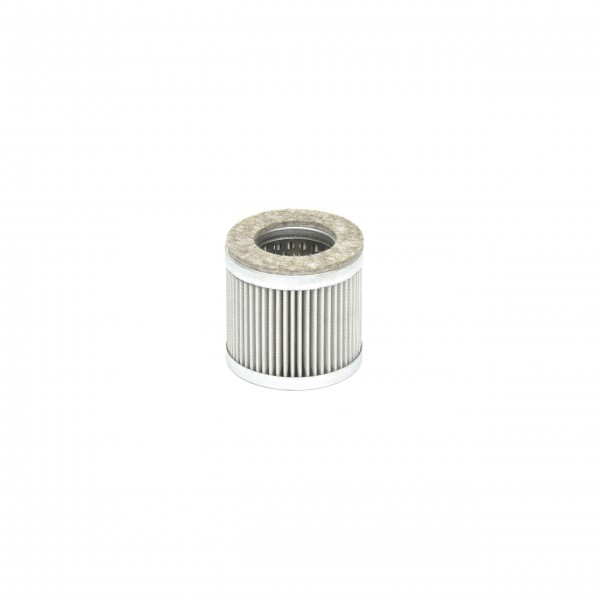# 90952900000 Filter CartridgeDelivery time: 1 - 3 business days

• 90952900000
Becker Original Filter Cartridge 90952900000 The filter medium's (paper) filtration... more
Product information "90952900000 Filter Cartridge"

Becker Original Filter Cartridge 90952900000
The filter medium's (paper) filtration efficiency is 99,97% at 0,3 µm. Filter cartridges made of paper can be cleaned by blowing through with clean dry compressed air. To ensure proper function of your Becker product, filter elements have to be cleaned and changed regularly according to the operation manual.

 Dimensions [mm]: 64-38-60 Filtration efficiency: 99,97% at 0,3 µm Filtration: Filter Cartridges Use of filter cartridge: T 3.25/40, DT/T/VT 4.25/4.40, Filter material: paperDT 3.25 (Index D) Order number: DT3.25-DDT 3.25 (Index E) Order number: DT3.25-EDT 3.25 (Index F) Order number: DT3.25-FDT 3.25 (Index G) Order number: DT3.25-GDT 3.40 (Index D) Order number: DT3.40-DDT 3.40 (Index E) Order number: DT3.40-EDT 3.40 (Index F) Order number: DT3.40-FDT 3.40 (Index G) Order number: DT3.40-G DT 4.25 K (Index A) Order number: DT4.25K-A DT 4.25 K (Index C) Order number: DT4.25K-CDT 4.25 K (single-phase AC) (Index A) Order number: DT4.25Ksingle-phaseAC-A DT 4.25 K/0-06 (Index A) Order number: DT4.25K0-06-ADT 4.25 K/0-06 (three-phase AC) (Index A) Order number: DT4.25K0-06three-phaseAC-A DT 4.25/0-80 (Index B) Order number: DT4.250-80-B DT 4.25/0-80 (Index C) Order number: DT4.250-80-C DT 4.40 K (Index A) Order number: DT4.40K-A DT 4.40 K (Index C) Order number: DT4.40K-CDT 4.40 K/0-06 (three-phase AC) (Index A) Order number: DT4.40K0-06three-phaseAC-ADT 4.40 K/5-63 (three-phase AC) (Index A) Order number: DT4.40K5-63three-phaseAC-ADT 4.40 K/5-63 (three-phase AC) (Index C) Order number: DT4.40K5-63three-phaseAC-C DT 4.40/0-80 (Index A) Order number: DT4.400-80-A DT 4.40/0-80 (Index C) Order number: DT4.400-80-C DX 4.25 K Order number: DX4.25K DX 4.25 K (Index C) Order number: DX4.25K-C DX 4.25/0-80 Order number: DX4.250-80 DX 4.25/0-80 (Index C) Order number: DX4.250-80-C DX 4.40 K Order number: DX4.40K DX 4.40 K (Index C) Order number: DX4.40K-C DX 4.40/0-80 Order number: DX4.400-80 DX 4.40/0-80 (Index C) Order number: DX4.400-80-CT 3.25 DSK (Index D) Order number: T3.25DSK-DT 3.25 DSK (Index E) Order number: T3.25DSK-ET 3.25 DSK (Index F) Order number: T3.25DSK-FT 3.25 DSK (Index G) Order number: T3.25DSK-GT 3.25 DSK, CSA (Index E) Order number: T3.25DSKCSA-ET 3.25 DSK, CSA (Index F) Order number: T3.25DSKCSA-FT 3.25 DSK, CSA (Index G) Order number: T3.25DSKCSA-GT 3.40 DSK (Index D) Order number: T3.40DSK-DT 3.40 DSK (Index E) Order number: T3.40DSK-ET 3.40 DSK (Index F) Order number: T3.40DSK-FT 3.40 DSK (Index G) Order number: T3.40DSK-G T 4.25 DSK (Index A) Order number: T4.25DSK-A T 4.25 DSK (Index C) Order number: T4.25DSK-C T 4.25 DSK (Index X) Order number: T4.25DSK-X T 4.25 DSK (Index Z) Order number: T4.25DSK-Z T 4.25 DV (Index A) Order number: T4.25DV-A T 4.25 DV (Index C) Order number: T4.25DV-C T 4.25 DV (Index X) Order number: T4.25DV-X T 4.25 DV (Index Z) Order number: T4.25DV-Z T 4.40 DSK (Index A) Order number: T4.40DSK-A T 4.40 DSK (Index C) Order number: T4.40DSK-C T 4.40 DSK (Index X) Order number: T4.40DSK-X T 4.40 DSK (Index Z) Order number: T4.40DSK-ZT 4.40 DSK, /-34 (Index A) Order number: T4.40DSK-34-AT 4.40 DSK, /-34 (Index C) Order number: T4.40DSK-34-CT 4.40 DSK, /-34 (Index X) Order number: T4.40DSK-34-XT 4.40 DSK, /-34 (Index Z) Order number: T4.40DSK-34-ZT 4.40 DV (Index A) Order number: T4.40DV-A T 4.40 DV (Index C) Order number: T4.40DV-C T 4.40 DV (Index X) Order number: T4.40DV-X T 4.40 DV (Index Z) Order number: T4.40DV-ZVT 3.25 (Index D) Order number: VT3.25-DVT 3.25 (Index E) Order number: VT3.25-EVT 3.25 (Index F) Order number: VT3.25-FVT 3.25 (Index G) Order number: VT3.25-GVT 3.40 (Index D) Order number: VT3.40-DVT 3.40 (Index E) Order number: VT3.40-EVT 3.40 (Index F) Order number: VT3.40-FVT 3.40 (Index G) Order number: VT3.40-G VT 4.40/0-65 (Index A) Order number: VT4.400-65-A VT 4.40/0-65 (Index C) Order number: VT4.400-65-C X 4.25 DSK Order number: X4.25DSKX 4.25 DSK (Index C) Order number: X4.25DSK-C X 4.40 DSK Order number: X4.40DSKX 4.40 DSK (Index C) Order number: X4.40DSK-CX 4.40 DV Order number: X4.40DVX 4.40 DV (Index C) Order number: X4.40DV-C
Viewed